Browse by Topic
Related Topics

# Energy Resource Dynamics with the New System Dynamics Library for SystemModeler

Explore the contents of this article with a free Wolfram SystemModeler trial. Wolfram SystemModeler ships with model libraries for a large selection of domains such as electronics, mechanics, and biochemistry. Now I am pleased to present a new library in the family, the SystemDynamics library by François E. Cellier and Stefan Fabricius. System dynamics, a methodology developed by Jay Forrester in the ’60s and ’70s, is well suited for understanding the dynamics of large-scale systems with diverse components. It has been famously applied by the Club of Rome to investigate the limits of human growth; other applications include production management, life sciences, and economics (some showcases of the methodology can be found here).

The dynamics of the many types of global energy resources are another good example of such a large and complex system, and are a recurring topic on politicians’ lips, so I thought that this could be a good starting point to explore the possibilities of this new library. The U.S. Department of Energy has for some time been working on a modeling system toward a better understanding of this problem, in order to let politicians make adequate legislation and policy decisions. Recreating their work (basically all energy demands in the US) is not possible within the scope of this blog post; I will however show an expandable framework for how one can do this, which will only focus on a single electricity producing plant and how it is affected by the global marketplace. But before we dig into more complex modeling, I’d like to start with a short introduction to the library and the methodology.The system dynamics methodology is used for modeling the continuous flow of mass and information, using in principle two types of objects: levels, which are quantities that can accumulate mass or information (state variables), and rates, which influence the accumulation/depletion of the levels (state derivatives). As an example: money is a typical level for which the inflow rate is the income and the outflow rate is the expense. The rates are in turn controlled by laundry lists (in the system dynamics terminology), which are sets of influencing factors that modify the rates. In this example these laundry lists would include for instance rent, food costs, and internet service. In the picture below this simple example is shown, where the interest is a function of the current money level; of course the rates can also be defined with a nonlinear relationship. The purple clouds are sources and sinks of mass used in the system dynamics methodology.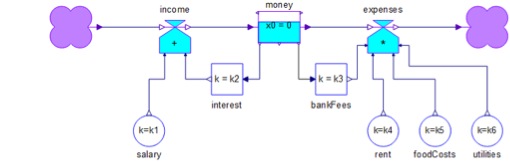So let’s explore how you can create models in order to understand energy resource dynamics using the SystemDynamics library, and use Wolfram|Alpha to get real data into the model.

### The model

As previously mentioned, the model contains a single electricity producing plant, and I have based this power plant on the newly finished Shoaiba power and desalination plant in Saudi Arabia. The desalination for salt water is not taken into account here. By connecting this production facility to the international fuel market and the regional electricity demand, we can start to look at questions such as, “How long will the plant be able to meet demand?” and “How is the price of electricity affected by high-efficiency turbines?”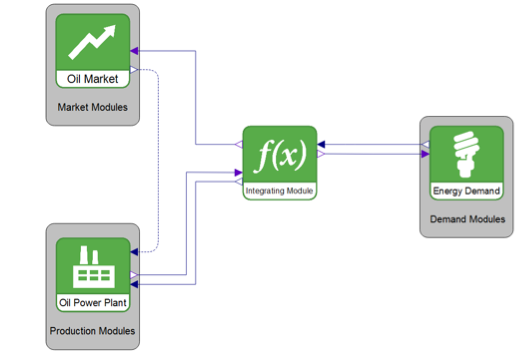The model consists of three primary modules: demand, market, and production. The overall structure, with an integrating module at its heart, allows for adding arbitrarily many other modules, that is, the integrating module connects all other modules together and routes the flows of mass or information to its designated module. In this case the integrating module is somewhat unnecessary, and adds extra equations, but it’s designed for showing how you can begin creating such a large system with a modular design.

In the market module, which supplies historic spot oil price data from Wolfram|Alpha, the data from Wolfram|Alpha is retrieved, processed, and exported to SystemModeler with just few lines of Mathematica code, starting with loading the Wolfram SystemModeler Link, which is needed for formatting the export, and also later on when I start interacting with the model in Mathematica.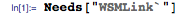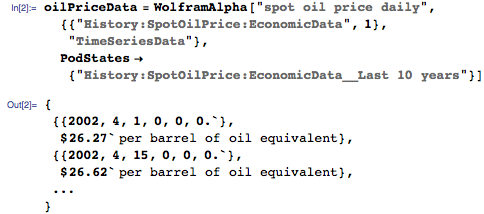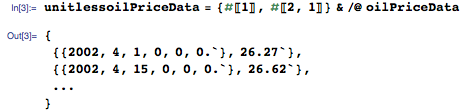Here I just convert the date strings to the number of days from the 1st of January 2002, so that it can be used in the model.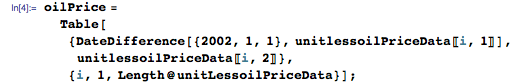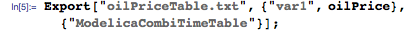The time series can now be used in SystemModeler trough the CombiTimeTable component in the Modelica standard library.

I’ve based the demand module on data from a Wolfram|Alpha query of Saudi Arabia’s electricity usage, where the yearly increase in electricity consumption is used as a base for generating the electricity needed in the demand module (a combination of sine and trapezoid functions is used for adding seasonal changes in between the data points).

The power plant in the production model uses gas turbines that burn oil in a combined cycle, which means that the exhaust gases are used in a secondary steam turbine for increased efficiency; these are known as combined-cycle gas turbines (CCGT), as compared to the older, less efficient open-cycle gas turbines (OCGT). The power plant consists of 14 CCGT units with maximal capacity of 400 MW, yielding a net capacity of 5.6 GW, spread out into 4 blocks with 4 or 3 gas turbines in each block. The production module is a fairly large hierarchical model scaling from a top level with controller and resource ordering logic down to a single gas turbine: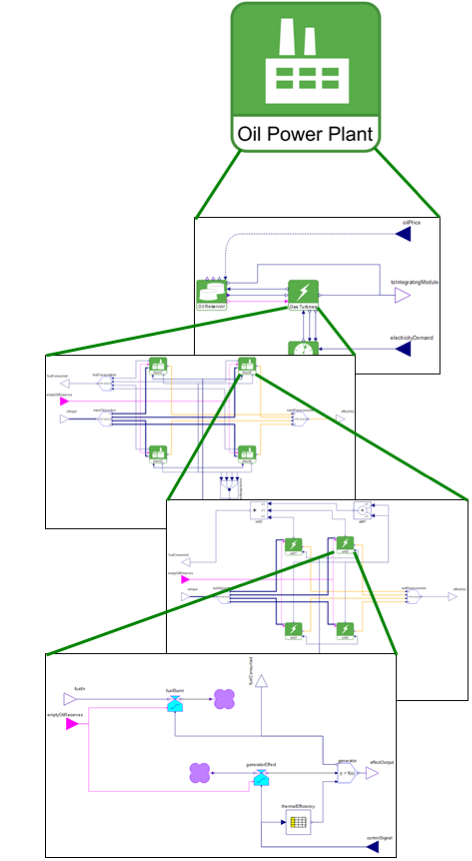At the turbine model level, the system dynamics rate classes are found, where the rate at which fuel is burned governs the reduction of the level in the oil reservoirs found higher up in the hierarchy. The oil consumption (the laundry list, in system dynamics terminology) calculations are based on the heat of combustion for oil, the thermal efficiency of the turbine, and the turbine load and effect.

The oil reservoir component of the power plant model can be found if we go back up the hierarchy a bit; here the amount of oil in storage is computed by taking into account the fuel burnt by the turbines and the tankers delivering new oil. Refueling the plant is modeled in a simplified fashion where the consumed amount is restored biweekly.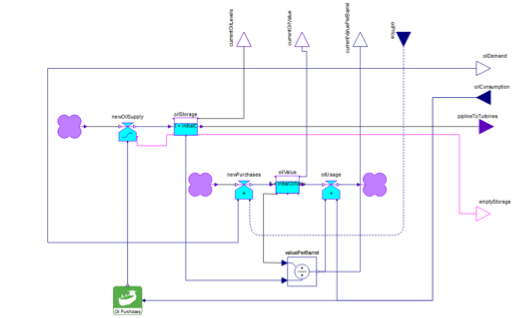The controller for the plant allows for a lowest turbine load of 40% and goes up with a load step size of 5% to the maximum load, where the turbine has the top efficiency. The startup time for a modern CCGT is about 30 minutes (from standstill to full load, with full efficiency); however, since the startup time is so small compared to the time step of the model (1 day), this effect is negligible and thus disregarded. The all-important thermal efficiency of the gas turbine varies as a function of the load, where the efficiency at a 40% turbine load is 50% and at a 100% turbine load is 60%, assuming a linear increase in efficiency between the two loads.

### Analyzing different scenarios

Let’s simulate the model and then analyze the results for a CCGT scenario for 10 years starting at April 1, 2002.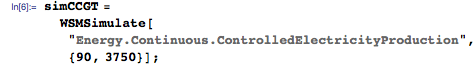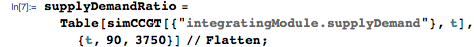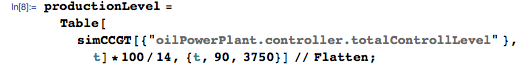For visualizing the analysis, I use the DateListPlot function.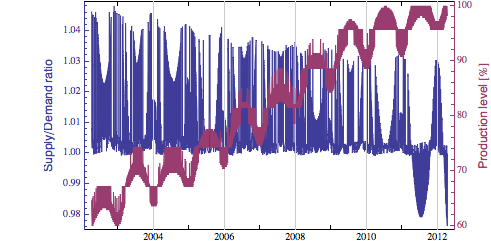Here it is apparent that the increase in electric demand reaches beyond the power output of the plant, which starts from about 60% load in 2002 and goes up to 100% in 2012. So there is a need to build even more power plants with this growth in demand; however, there are of course more power plants out there in real life to pick up the slack, but the increase in demand is quite massive.

The price for the electricity is largely due to the fuel costs, which were retrieved from Wolfram|Alpha and used in the model.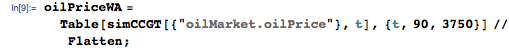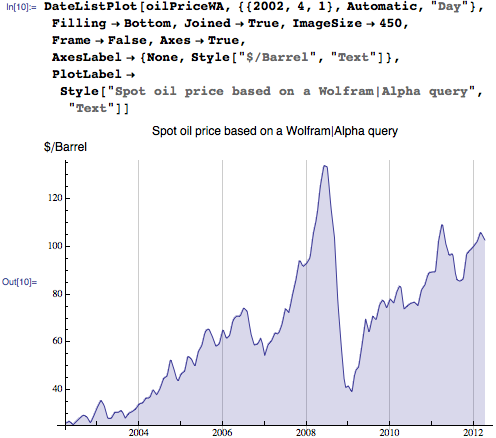Before I move further with the analyses of the system, let’s define another scenario where the simpler open cycle gas turbine technology is used, assuming an efficiency of 35% and 42% for 40% and 100% loads, respectively. The simulation call to SystemModeler is similar to the first scenario; I just define a set of new parameters for this scenario.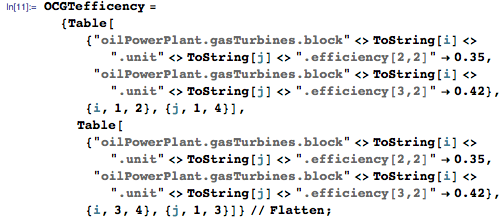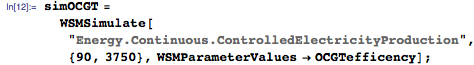Since the turbines are not run at their full load at all times, the actual efficiency where the demand and controller schemes are also taken into account is more interesting than the mere theoretical efficiency. For this comparison I will look at the entire 10-year time scale: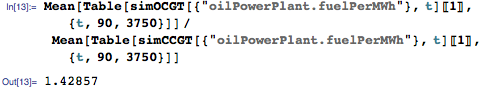So for this scenario and these assumptions, the CCGT is on average 43% more efficient than the OCGT turbine. Visualizing these differences throughout the 10-year period is easily done, and by using the DatePlus function to create the date strings, we get a nice x axis in the figure.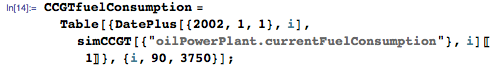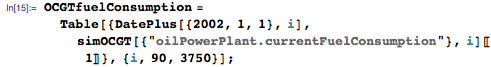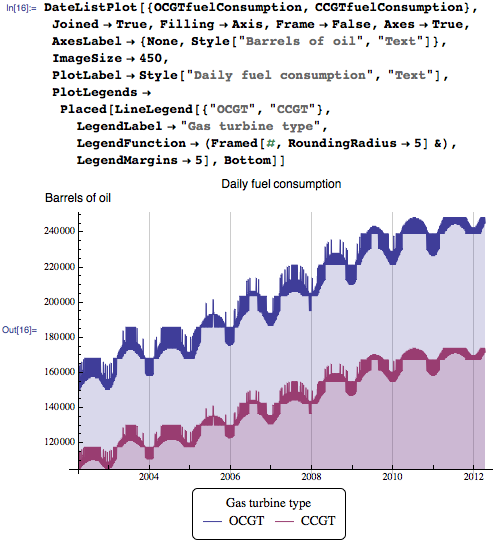The cost of producing electricity is largely defined by the fuel costs, and with the market module, the associated fuel costs for the plant’s production can be calculated. Fuel price spikes can strike hard at the economy of, for instance, private households and energy demand industries. Spikes effectively lower their ability to consume other goods and services in a larger complex model where the consumers’ ability to consume affects the industry demand. These feedback loops are at the heart of the system and are important structural aspects in many system dynamics applications.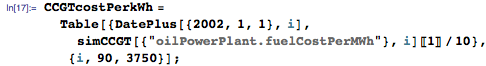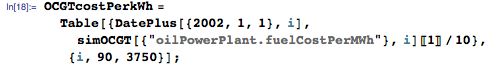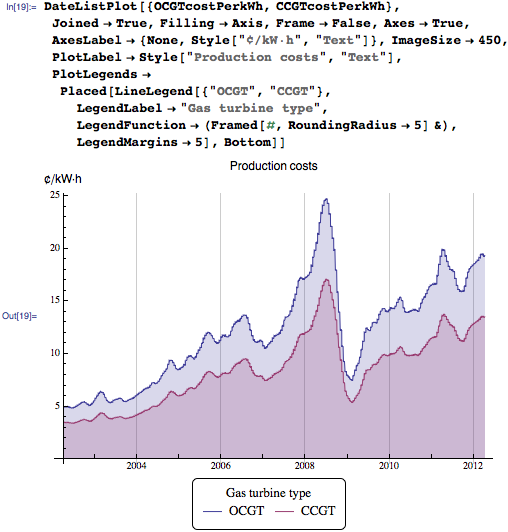Due to the rapid increase in the spot oil price during the last 10 years, the fuel part of the production costs of electricity increases quite rapidly, especially for the OCGT type, starting at 5¢ in 2002 and topping off at 25¢ in mid-2008. Note that these costs are affected by the heat of combustion of the fuel oil (in this case it’s assumed to be 139 500 Btu/gallon), and that the fuel price used here is the spot oil price. Moreover, the costs are also dependent on the scenario specifications.